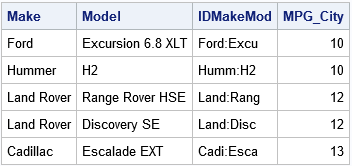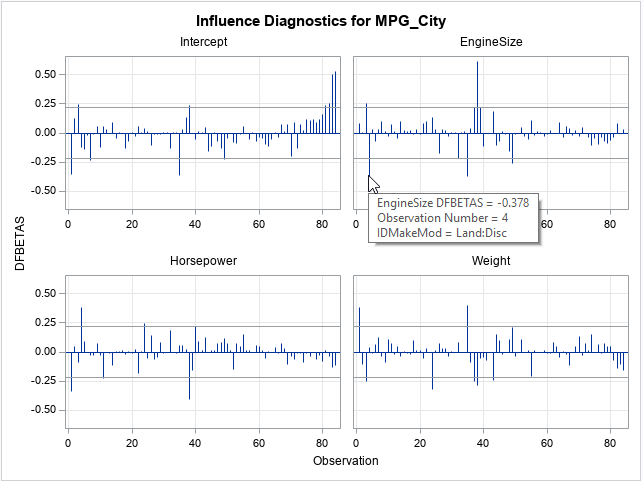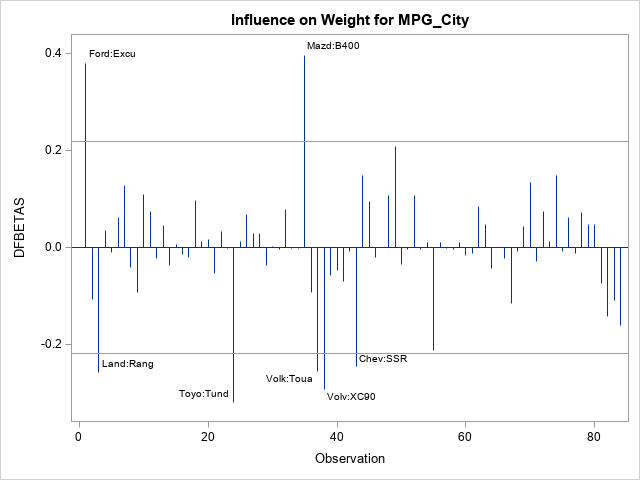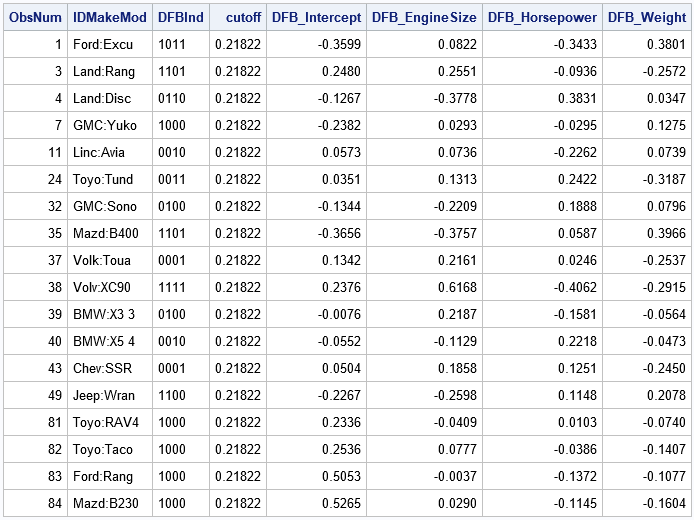My article about deletion diagnostics investigated how influential an observation is to a least squares regression model. In other words, if you delete the i_th observation and refit the model, what happens to the statistics for the model? SAS regression procedures provide many tables and graphs that enable you to examine the influence of deleting an observation. For example:

• The DFBETAS are statistics that indicate the effect that deleting each observation has on the estimates for the regression coefficients.
• The DFFITS and Cook's D statistics indicate the effect that deleting each observation has on the predicted values of the model.
• The COVRATIO statistics indicate the effect that deleting each observation has on the variance-covariance matrix of the estimates.

These observation-wise statistics are typically used for smaller data sets (n ≤ 1000) because the influence of any single observation diminishes as the sample size increases. You can get a table of these (and other) deletion diagnostics by using the INFLUENCE option on the MODEL statement of PROC REG in SAS. However, because there is one statistic per observation, these statistics are usually graphed. PROC REG can automatically generate needle plots of these statistics (with heuristic cutoff values) by using the PLOTS= option on the PROC REG statement.

This article describes the DFBETAS statistic and shows how to create graphs of the DFBETAS in PROC REG in SAS. The next article discusses the DFFITS and Cook's D statistics. The COVRATIO statistic is not as popular, so I won't say more about that statistic.

### DFBETAS: How the coefficient estimates change if an observation is excluded

The documentation for PROC REG has a section that describes the influence statistics, which is based on the book Regression Diagnostics by Belsley, Kuh, and Welsch (1980, p. 13-14). Among these, the DFBETAS statistics are perhaps the easiest to understand. If you exclude an observation from the data and refit the model, you will get new parameter estimates. How much do the estimates change? Notice that you get one statistic for each observation and also one for each regressor (including the intercept). Thus if you have n observations and k regressors, you get nk statistics.

Typically, these statistics are shown in a panel of k plots, with the DFBETAS for each regressor plotted against the observation number. Because "observation number" is an arbitrary number, I like to sort the data by the response variable. Then I know that the small observation numbers correspond to low values of the response variable and large observation numbers correspond to high values of the response variable. The following DATA step extracts a subset of n = 84 vehicles from the Sashelp.Cars data, creates a short ID variable for labeling observations, and sorts the data by the response variable, MPG_City:

```data cars; set sashelp.cars; where Type in ('SUV', 'Truck'); /* make short ID label from Make and Model values */ length IDMakeMod \$20; IDMakeMod = cats(substr(Make,1,4), ":", substr(Model,1,5)); run;   proc sort data=cars; by MPG_City; run;   proc print data=cars(obs=5) noobs; var Make Model IDMakeMod MPG_City; run;```The first few observations are shown. Notice that the first observations correspond to small values of the MPG_City variable. Notice also a short label (IDMakeMod) identifies each vehicle.

There are two ways to generate the DFBETAS statistics: You can use the INFLUENCE option on the MODEL statement to generate a table of statistics, or you can use the PLOTS=DFBETAS option in the PROC REG statement to generate a panel of graphs. The following call to PROC REG generates a panel of graphs. The IMAGEMAP=ON option on the ODS GRAPHICS statement enables you to hover the mouse pointer over an observation and obtain a brief description of the observation:

```ods graphics on / imagemap=on; /* enable data tips (tooltips) */ proc reg data=Cars plots(only) = DFBetas; model MPG_City = EngineSize HorsePower Weight; id IDMakeMod; run; quit; ods graphics / imagemap=off;```The panel shows the influence of each observation on the estimates of the four regression coefficients. The statistics are standardized so that all graphs can use the same vertical scale. Horizontal lines are drawn at ±2/sqrt(n) ≈ 0.22. Observations are called influential if they have a DFBETA statistic that exceeds that value. The graph shows a tool tip for one of the observations in the EngineSize graph, which shows that the influential point is observation 4, the Land Rover Discovery.

Each graph reveals a few influential observations:

• For the intercept estimate, the most influential observations are numbers 1, 35, 83, and 84.
• For the EngineSize estimates, the most influential observations are numbers 4, 35, and 38.
• For the Horsepower estimates, the most influential observations are numbers 1, 4, and 38.
• For the Weight estimates, the most influential observations are numbers 1, 24, 35, and 38.

Notice that several observations (such as 1, 35, and 38) are influential for more than one estimate. Excluding those observations causes several parameter estimates to change substantially.

### Labeing the influential observations

For me, the panel of graphs is too small. I found it difficult to hover the mouse pointer exactly over the tip of a needle in an attempt to discover the observation number and name of the vehicle. Fortunately, if you want details like that, PROC REG supplies options that make the process easier. If you don't have too many observations, you can add labels to the DFBETAS plots by using the LABEL suboption. To plot each graph individually (instead of in a panel), use the UNPACK suboption, as follows:

```proc reg data=Cars plots(only) = DFBetas(label unpack); model MPG_City = EngineSize HorsePower Weight; id IDMakeMod; quit;```The REG procedure creates four plots, but only the graph for the Weight variable is shown here. In this graph, the influential observations are labeled by the IDMakeMod variable, which enables you to identify vehicles rather than observation numbers. For example, some of the influential observations for the Weight variable are the Ford Excursion (1), the Toyota Tundra (24), the Mazda B400 (35), and the Volvo XC90 (38).

### A table of influential observations

If you want a table that displays the most influential observations, you can use the INFLUENCE option to generate the OutputStatistics table, which contains the DFBETAS for all regressors. You can write that table to a SAS data set and exclude any that do not have a large DFBETAS statistic, where "large" means the magnitude of the statistic exceeds 2/sqrt(n), where n is the sample size. The following DATA step filters the observations and prints only the influential ones.

```ods exclude all; proc reg data=Cars plots=NONE; model MPG_City = EngineSize HorsePower Weight / influence; id IDMakeMod; ods output OutputStatistics=OutputStats; /* save influence statistics */ run; quit; ods exclude none;   data Influential; set OutputStats nobs=n; array DFB[*] DFB_:; cutoff = 2 / sqrt(n); ObsNum = _N_; influential = 0; DFBInd = '0000'; /* binary string indicator */ do i = 1 to dim(DFB); if abs(DFB[i])>cutoff then do; /* this obs is influential for i_th regressor */ substr(DFBInd,i,1) = '1'; influential = 1; end; end; if influential; /* output only influential obs */ run;   proc print data=Influential noobs; var ObsNum IDMakeMod DFBInd cutoff DFB_:; run;```The DFBInd variable is a four-character binary string that indicates which parameter estimates are influenced by each observation. Some observations are influential only for one coefficient; others (1, 3, 35, and 38) are influential for many variables. Creating a binary string for each observation is a useful trick.

By the way, did you notice that the name of the statistic ("DFBETAS") has a large S at the end? Until I researched this article, I assumed it was to make the word plural since there is more than one "DFBETA" statistic. But, no, it turns out that the S stands for "scaled." You can define the DFBETA statistic (without the S) to be the change in parameter estimates bb(i), but that statistic depends on the scale of the variables. To standardize the statistic, divide by the standard error of the parameter estimates. That scaling is the reason for the S as the end of DFBETAS. The same is true for the DFFITS statistic: S stands for "scaled."

The next article describes how to create similar graphs for the DFFITS and Cook's D statistics.

---------------

### DFFITS: How the predicted values change if an observation is excluded

The DFFITS statistic measures, for each observation, how the predicted value at that observation changes if you exclude the observation and refit the model.

### Cook's D: How the sum of the predicted values change if an observation is excluded

Cook's distance (D) statistic measures, for each observation, the sum of the differences in the predicted values (summed over all observations) if you exclude the observation and refit the model.

The post Influential observations in a linear regression model: The DFBETAS statistics appeared first on The DO Loop.